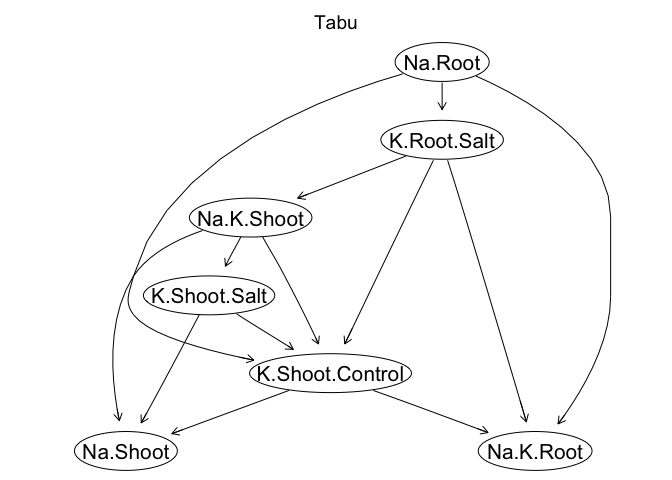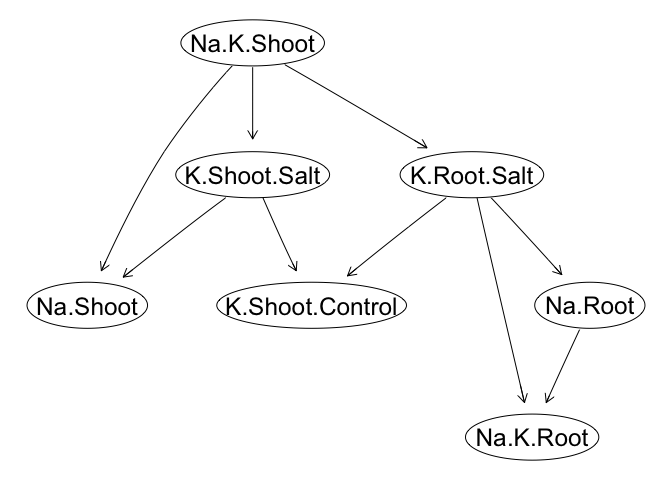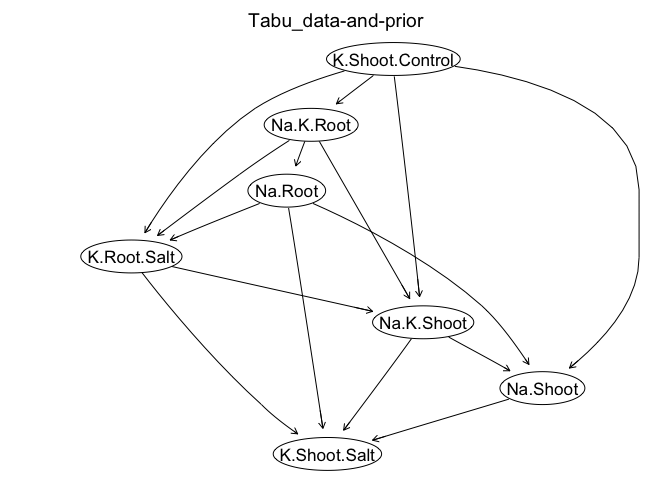# Bayesian Network

## Introduction

A Bayesian network (BN) describes the joint probability distribution of random variables through their conditional dependencies/independencies and represents as a graphical model (directed acyclic graph)(Scutari and Denis 2014). Thus, a BN provides an approach to decipher the probabilistic dependencies among variables of interest and has been applied in plant and animal genetics (Morota et al. 2012; Yu et al. 2019).

In the following examples, we use the bnlearn package (Scutari 2010) to infer the BN of seven rice phenotypes including Na+, K+, and Na+: K+ content in roots and shoots (Yu et al. 2019). The rice genotypes are from the Rice Diversity Panel 1 (Zhao et al. 2011). We will use two different approaches to infer BN: 1) data-driven approach and 2) the combination of the data-driven approach and prior knowledge. You can download the rice dataset here using wget or DownGit.

# install.packages("bnlearn")
library(bnlearn)
# install.packages("cowplot")
library(cowplot)
# install.packages("ggplot2")
library(ggplot2)
# install.packages("gaston")
library(gaston)
# install.packages("BiocManager")
# BiocManager::install("Rgraphviz")
library(Rgraphviz)
# install.packages("devtools")
library(devtools)
# install_github("QuantGen/MTM")
library(MTM)

## Data import

# rm(list = ls())

# Import phenotypes
pheno <- read.csv("salt_phenotypes.csv", row.names = 1)
dim(pheno) # 385   7
##  385   7
pheno <- na.omit(pheno) # remove NAs
dim(pheno) # 366   7
##  366   7
head(pheno)
##           Na.K.Shoot  Na.Shoot K.Shoot.Salt K.Shoot.Control Na.K.Root   Na.Root
## NSFTV_1     217.9371 0.1949661    0.8960666       0.9117648  500.9474 0.2933769
## NSFTV_10    137.4868 0.1250447    0.9109011       0.9235181  427.0097 0.2970459
## NSFTV_100   165.0296 0.1667733    1.0206480       1.0795380  454.0931 0.3127060
## NSFTV_101   146.2632 0.1574553    1.1010989       1.0868638  466.6072 0.3068186
## NSFTV_102   187.6632 0.1537053    0.8338489       0.9042839  522.5943 0.4205350
## NSFTV_103   119.3382 0.1102053    0.9420989       1.0959358  383.7196 0.2723217
##           K.Root.Salt
## NSFTV_1     0.6045812
## NSFTV_10    0.7066461
## NSFTV_100   0.6728234
## NSFTV_101   0.6662068
## NSFTV_102   0.8076836
## NSFTV_103   0.7132342
# Import genotype
geno <- read.bed.matrix("sativas413")
## Reading sativas413.fam
## ped stats and snps stats have been set.
## 'p' has been set.
## 'mu' and 'sigma' have been set.
dim(geno) # 413 36901
##    413 36901
## Subset accesions with both genotype and phenotype records
geno@ped$id <- paste0("NSFTV_", geno@ped$id)
geno <- geno[geno@ped$id %in% row.names(pheno), ] dim(geno) # 363 36901 ##  363 36901 pheno <- pheno[match(geno@ped$id, row.names(pheno)), ]
dim(pheno) # 363   7
##  363   7
## Check if the ids of genotypes and phenotypes are identical
table(geno@ped$id == rownames(pheno)) ## ## TRUE ## 363 ## Construct a genomic relationship matrix # Quality control of genotypes geno <- select.snps(geno, c(maf > 0.05 & callrate > 0.9)) dim(geno) # 363 29499 ##  363 29499 # Construct a genomic relationship matrix grm <- GRM(geno) dim(grm) # 363 363 ##  363 363 ## Fit a multivariate mixed model to obtain estimated breeding values (EBV) # Define the MTM_func using the MTM Package MTM_func <- function(Y, G, nTrait, nIter, burnIn, thin, prefix) { library(MTM) set.seed(007) # reproducible results MTM ( Y = Y, K = list ( list ( K = G, COV = list ( type = 'UN', df0 = nTrait, S0 = diag (nTrait) ) ) ), resCov = list ( type = 'UN', S0 = diag (nTrait), df0 = nTrait), nIter = nIter, burnIn = burnIn, thin = thin, saveAt = prefix) } Y <- scale(pheno, center = TRUE, scale = TRUE) G <- grm nTrait <- ncol(Y) MTM_fit <- MTM_func(Y = Y, G = G, nTrait = nTrait, nIter = 1200, burnIn = 200, thin = 5, prefix = 'MTM_fit') ## Check Gibbs samples list.files(pattern = 'MTM_fit') ## Retrieve estimates str(MTM_fit) BV <- MTM_fit$K[]$U # estimated breeding values dim(BV) # 363 7 head(BV) colnames(BV) <- colnames(pheno) BV <- as.data.frame(BV) head(BV) ## Structure learning using EBV ## Check strength and direction in BN check_boot <- function(boot, Strength_Thres, Direction_Thres) { boot[(boot$strength >= Strength_Thres) & (boot\$direction > Direction_Thres),]
}

## Remove the dependence in EBV with Cholesky Decomposition

Theoretically, BN learning algorithms assume sample independence. However, the genomic relationship matrix introduces dependencies among EBV, which may act as a confounder. We can transform EBV to obtain adjusted EBV by eliminating the dependencies. We can decompose $$\mathbf{G}$$ into its Cholesky factors $$\mathbf{G} = \mathbf{L} \mathbf{L'}$$. Here, $$\mathbf{L}$$ is an $$n \times n$$ lower triangular matrix. For a single trait we can remove the dependancy from the BV $$\mathbf{u}$$ and yield the adjusted breeding values $$\mathbf{u^*}$$ by $$\mathbf{u^*} = \mathbf{L^{-1}} \mathbf{u}$$. When we have $$t$$ traits, the dimension of $$\mathbf{u}$$ becomes $$(n \times t) \times 1$$ and $$\mathbf{u^*} = \mathbf{M^{-1}} \mathbf{u}$$, where $$\mathbf{M^{-1}} = \mathbf{I_{(n \times t) \times (n \times t)}} \otimes \mathbf{L^{-1}}$$.

Linv <- solve(t(chol(grm)))
Minv <- kronecker(diag(7), Linv)
BV_adj <- matrix(Minv %*% c(as.matrix(BV)), nrow = 363, ncol = 7)
BV_adj <- as.data.frame(BV_adj)

### Structure learning: data-driven approach

# Score-based algorithm: Tabu Search
graphviz.plot(tabu_simple, main = "Tabu", shape = "ellipse", layout = "dot")# Model Averaging with 500 bootstrap samples to account for uncertainty
set.seed(007)
boot_tabu <- boot.strength (BV_adj, algorithm = "tabu", R = 500)
# Check strength (>= 0.8) and direction (> 0.5)
check_boot(boot_tabu, 0.8, 0.5)
##            from              to strength direction
## 1    Na.K.Shoot        Na.Shoot    1.000 0.8350000
## 2    Na.K.Shoot    K.Shoot.Salt    1.000 0.6230000
## 6    Na.K.Shoot     K.Root.Salt    0.960 0.5093750
## 14 K.Shoot.Salt        Na.Shoot    0.956 0.7677824
## 15 K.Shoot.Salt K.Shoot.Control    1.000 0.9710000
## 35      Na.Root       Na.K.Root    1.000 0.6390000
## 40  K.Root.Salt K.Shoot.Control    0.950 0.9957895
## 41  K.Root.Salt       Na.K.Root    1.000 0.7620000
## 42  K.Root.Salt         Na.Root    1.000 0.6620000
# Average bootstrap samples use threshold = 0.8
ave_model_tabu <- averaged.network(boot_tabu, threshold = 0.8)
graphviz.plot(ave_model_tabu, shape = 'ellipse')### Structure learning: data + prior knowledge

If prior knowledge regarding the network structure is available, it is possible to incorporate this into the process of structure learning. We can add and eliminate the edge using arguments of whitelist and blacklist, accordingly.

# Score-based algorithm: Tabu Search
graphviz.plot(tabu_simple, main = "Tabu", shape = "ellipse", layout = "dot")# Incorporate subjective prior
whtlist <- c(from = 'Na.K.Root', to = 'Na.K.Shoot')
blklist <- data.frame(from = c("K.Shoot.Salt", "K.Shoot.Control" ),
to = c("K.Shoot.Control", "K.Shoot.Salt"))
# Data + subjective prior
tabu_blk <- tabu(BV_adj, whitelist = whtlist, blacklist = blklist)
graphviz.plot(tabu_blk, main = "Tabu_data-and-prior", shape = "ellipse",
layout = "dot")## Available BN learning algorithms in the bnlearn package

In the above examples, we used the score-based algorithm Tabu Search for structure learning. Alternatively, you can use other algorithms by replacing the tabu() function and the algorithm = "tabu" argument in the boot.strength() function with the followings. The details can be found in the help page of bnlearn.

• Score-based structure learning algorithms:
• hc: Hill Climbing (HC)
• tabu: Tabu Search (Tabu)
• Constraint-based structure learning algorithms:
• pc.stable : the first practical application of the IC algorithm
• gs: Grow-Shrink (GS)
• iamb: Incremental Association Markov Blanket (IAMB)
• fast.iamb: Fast Incremental Association (Fast-IAMB)
• inter.iamb: Interleaved Incremental Association (Inter-IAMB)
• mmpc: Max-Min Parents & Children (MMPC)
• si.hiton.pc: Semi-Interleaved Hiton-PC (SI-HITON-PC)
• Hybrid structure learning algorithms:
• mmhc: Max-Min Hill Climbing (MMHC)
• rsmax2: General 2-Phase Restricted Maximization (RSMAX2)